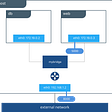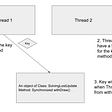# Big-O Notation and Time Complexity Explained!

Originally published on Hashnode.

Table of content:

• Introduction
• What is Big-O Notation and Time Complexity?
• Why should I care?
• How to calculate it?
• Common complexity classes

# Introduction

The role of software engineers is to solve real world problems by designing algorithms. Coming up with a solution is often easy but that is not the challenge, rather it is coming up with one that is optimal.

In what terms exactly?

In time execution, required space, code readability…

It all depends on the given situation, you get the point.

And for that, familiarity with Time Complexity is required.

# What is Big-O Notation and Time Complexity?

## Time Complexity:

It is an estimation of the efficiency of an algorithm for a given input.

## Big-O Notation:

It is a notation for Time Complexity, denoted O(something).

Also known as Bachmann-Landau notation or asymptotic notation for readers who are interested in math.

Pronounced: O of something, Order of something or Big-O of something.

Whereas “something” is a mathematical function of n.

n is the input size. For example, if the input is a string, n would be the length of it (number of characters). And if it is an array, it is the number of elements in it.

I recommend Wikipedia for a detailed definition.

# Why should I care?

Most interviews (if not all) for software engineering roles test your problem solving AND algorithm optimization skills; how to come up with an efficient solution and how to enhance it further.

With Time Complexity you will have solid knowledge of how efficient your algorithms are before implementing them!

You will also have an idea of which approach to take through the size of the input.

Additionally, if you’re into competitive programming, this will help you greatly with avoiding Time Limit Exceeded flags.

# So how do I calculate it?

Now this is the fun part!

## Loops

What makes an algorithm so slow most of the time is having many nested loops; the more nested loops, the slower:

If there are k nested loops, the Time Complexity is O(n^k)

The following code has O(n) complexity:

## Order of magnitude

As we mentioned previously, a Time Complexity is an estimation of an algorithm, which means it does not provide us with the exact number of times the code inside of our loop is executed.

So what does it provide us with?

It provides us with the magnitude.

For example:

• O(2n + 1) is O(n)
• O(1000n +300) is O(n)
• O(5n²) is O(n²)

And so on and so forth…

## Phases

A phase is a single loop in a code.

For example:

So how do we calculate the time complexity of such code with multiple phases?

We take the largest time complexity of a single phase.

Why?

Remember, that we’re looking for an estimation and since the phase with the largest time complexity is the slowest to execute, its complexity is approximately the overall time complexity of our code.

To follow up with the previous code snippet, the time complexity of it is the 2nd phase’s time complexity, which is O(n*m)

Sometimes, time complexity depends on additional factors to the input size n, like for example m in the previous example.

## Common complexity classes

These are some complexities you will most likely frequent:

• Constant-time algorithm O(1): the function (which is f(n) = 1) does not depend on n; the input size does not affect execution time. We commonly see this with mathematical formulas.
• Linear algorithm O(n): the execution time grows proportionately as the input size grows as well; f(n) = n. When a certain data manipulation is needed, this is often the best solution since it traverses the input only once!
• Quadratic algorithm O(n²): contains two nested loops. Usually goes through the input twice. Same goes for the cubic algorithm O(n³) that has three nested loops in turn; going through the input three times.
• Logarithmic algorithm O(log(n)): it is logarithmic because at each step, the input size is halved, log(n) thus is the number of times n must be divided to get 1.
• O(n!): it often means that the algorithm goes through all possible permutations of the input.

That was it ladies and gentlemen, I hope you enjoyed the article!

Any feedback or constructive critique is warmly welcomed and appreciated, so please tell me what you think in the comments so I can better the quality. Thanks for reading ❤️!

Have a nice one!

--

--

--

## More from Mohamed El Amine Yamani

Just a front-end guy and a bookworm who loves to share what he is learning.

Love podcasts or audiobooks? Learn on the go with our new app.

## Docker Networking(Bridge-Network)## Build or Buy? — How You decide## Multi-threading (iii) dealing with concurrency issue## Core Web Vitals explained with GIFs## The Best and Worst Catan Board Setups## Getting started with the data-driven way for SaaS product management.## Blogger Header Image Amp Code | Template Amp Header Issu Fix ?## Mohamed El Amine Yamani

Just a front-end guy and a bookworm who loves to share what he is learning.

## More from Medium